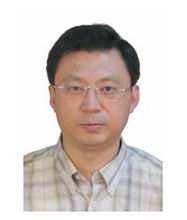Inverse Problems: Analysis, Computation, and Applications
Speaker
Prof. Gang Bao
School of Mathematical Sciences, Zhejiang University
Abstract

Inverse problems arise in diverse areas of industrial and military applications, such as nondestructive testing, seismic imaging, submarine detections, near-field and nano optical imaging, and medical imaging. A model problem in wave propagation is concerned with a plane wave incident on a medium enclosed by a bounded domain. Given the incident field, the direct problem is to determine the scattered field for the known scatterer. The inverse problem is to determine the scatterer from the boundary measurements of near field currents densities. Although this is a classical problem in mathematical physics, mathematical issues and numerical solution of the inverse problems remain to be challenging since the problems are nonlinear, large-scale, and most of all ill-posed! The severe ill-posedness has thus far limited in many ways the scope of inverse problem methods in practical applications. In this talk, the speaker will first introduce general inverse problems and discuss the state of the arts of the inverse problems. Our recent progress in mathematical analysis and computational studies of the inverse boundary value problems will be reported. Several classes of inverse problems will be studied, including inverse medium problems, inverse source problems, inverse obstacle problems, and inverse waveguide problems. A novel stable continuation approach based on the uncertainty principle will be presented. By using multi-frequency or multi-spatial frequency boundary data, our approach is shown to overcome the ill-posedness for the inverse problems. New stability results and techniques for the inverse problems will be presented. Related topics will be highlighted.